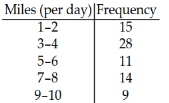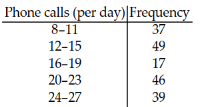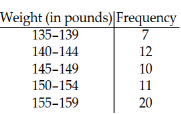/
/
/
3 Find the Weighted Mean MULTIPLE CHOICE. Choose the one alternative
Not my Question
Flag Content

# Question : 3 Find the Weighted Mean MULTIPLE CHOICE. Choose the one alternative : 2075521

3 Find the Weighted Mean

MULTIPLE CHOICE. Choose the one alternative that best completes the statement or answers the question.

Provide an appropriate response.

1) A student receives test scores of 62, 83, and 91. The student?s final exam score is 88 and homework score is 76.

Each test is worth 20% of the final grade, the final exam is 25% of the final grade, and the homework grade is 15% of the final grade. What is the student?s mean score in the class?

A) 80.6

B) 76.6

C) 90.6

D) 85.6

2) Grade points are assigned as follows: A = 4, B = 3, C = 2, D = 1, and F = 0. Grades are weighted according to credit hours. If a student receives an A in a four-credit class, a D in a two-credit class, a B in a three-credit class and a C in a three-credit class, what is the student?s grade point average?

A) 2.75

B) 1.75

C) 2.50

D) 3.00

4 Find the Mean of Frequency Distribution

MULTIPLE CHOICE. Choose the one alternative that best completes the statement or answers the question.

Approximate the mean of the frequency distribution.

1)A) 5

B) 4

C) 6

D) 15

2)A) 18

B) 17

C) 16

D) 19

E) 38

3)A) 149

B) 147

C) 151

D) 12

## Solution 5 (1 Ratings )

Solved
Statistics 5 Days Ago 30 Views